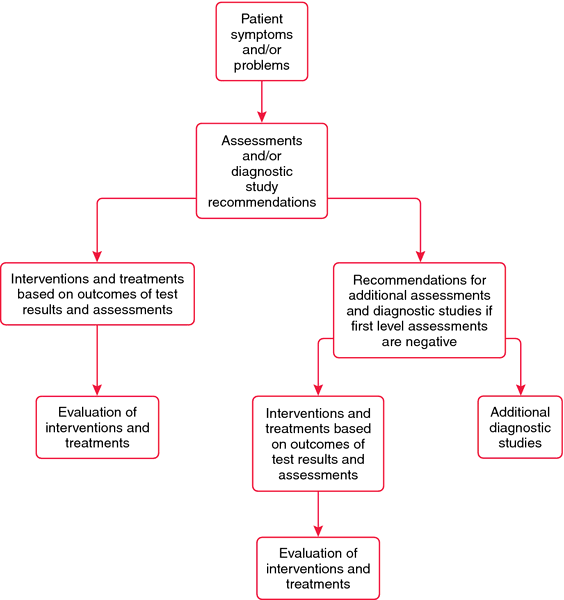algorithm

(redirected from Algorithm segment)
Also found in: Dictionary, Thesaurus, Financial, Encyclopedia.

algorithm

[al´go-rithm]
1. a series of algebraic equations.
2. a logical progression that is programmed for a computer.
3. a model for making decisions.Algorithm. Model of a decision algorithm. ACC/AHA Guidelines for the Management of Patients with Unstable Angina and Non-ST Segment Elevation Myocardial Infarction. JACC 2000, 36: 970-1062. Copyright 2000, by the American College of Cardiology and American Heart Association. Permission granted for one time use. Further reproduction is not permitted without permission of the ACC/AHA.

al·go·rithm

(al'gō-ridhm),
A systematic process consisting of an ordered sequence of steps, each step depending on the outcome of the previous one. In clinical medicine, a step-by-step protocol for management of a health care problem; in computed tomography, the formulas used to calculate the final image from the transmitted x-ray data.
[Mediev. L. algorismus, after Muhammad ibn-Musa al-Khwarizmi, Arabian mathematician, + G. arithmos, number]

algorithm

(1) A sequential procedure for solving a mathematical problem.
(2) A step-by-step procedure for reaching a decision when choosing among multiple alternative options, linked to each other by a decision tree.

algorithm

Decision-making A logical set of rules for solving a specific problem, which assumes that all of the data is objective, that there are a finite number of solutions to the problem, and that there are logical steps that must be performed to arrive at each of those solutions NIHspeak A step-by-step procedure for solving a problem; a formula. See Back-propagation, Critical pathway, Genetic algorithm, Risk of ovarian cancer algorithm.

al·go·rithm

(al'gŏr-idhm)
1. A process consisting of steps, each depending on the outcome of the previous one.
2. clinical medicine A step-by-step protocol for management of a health care problem.
3. computed tomography The formulas used for calculation of the final image from the x-ray transmission data.
[Mediev. L. algorismus, after Muhammad ibn-Musa al-Khwarizmi, Persian mathematician, + G. arithmos, number]

al·go·rithm

(al'gŏr-idhm)
A systematic process consisting of an ordered sequence of steps, each step depending on the outcome of the previous one.
[Mediev. L. algorismus, after Muhammad ibn-Musa al-Khwarizmi, Persian mathematician, + G. arithmos, number]
Site: Follow: Share:
Open / Close